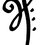# easy multiplication

Multiply any two numbers from 11 to 20 in your head:-


Multiply any two numbers from 11 to 20 in your head Take 15 x 13 for example… Place the larger no. first in your mind and then do something like this.

Take the larger no on the top and the second digit of the smaller no. in the bottom. 15 3 The rest is quite simple. Add 15+3 = 18 . Then multiply it by 10. In this example – 18 x 10 = 180 … Now multiply the second digit of both the no.s (ie; 5 x 3 = 15) Now add 180 + 15. Here is the answer 180 + 15 = 195 . Think over it. This is a simple trick. It will help you a lot.please comment after readingNote by Vishwathiga Jayasankar
6 years, 9 months ago

This discussion board is a place to discuss our Daily Challenges and the math and science related to those challenges. Explanations are more than just a solution — they should explain the steps and thinking strategies that you used to obtain the solution. Comments should further the discussion of math and science.

When posting on Brilliant:

• Use the emojis to react to an explanation, whether you're congratulating a job well done , or just really confused .
• Ask specific questions about the challenge or the steps in somebody's explanation. Well-posed questions can add a lot to the discussion, but posting "I don't understand!" doesn't help anyone.
• Try to contribute something new to the discussion, whether it is an extension, generalization or other idea related to the challenge.

MarkdownAppears as
*italics* or _italics_ italics
**bold** or __bold__ bold
- bulleted- list
• bulleted
• list
1. numbered2. list
1. numbered
2. list
Note: you must add a full line of space before and after lists for them to show up correctly
paragraph 1paragraph 2

paragraph 1

paragraph 2

[example link](https://brilliant.org)example link
> This is a quote
This is a quote
    # I indented these lines
# 4 spaces, and now they show
# up as a code block.

print "hello world"
# I indented these lines
# 4 spaces, and now they show
# up as a code block.

print "hello world"
MathAppears as
Remember to wrap math in $$ ... $$ or $ ... $ to ensure proper formatting.
2 \times 3 $2 \times 3$
2^{34} $2^{34}$
a_{i-1} $a_{i-1}$
\frac{2}{3} $\frac{2}{3}$
\sqrt{2} $\sqrt{2}$
\sum_{i=1}^3 $\sum_{i=1}^3$
\sin \theta $\sin \theta$
\boxed{123} $\boxed{123}$

Sort by:

12 x 13 = 156. Nice little trick. Every bit of knowledge counts. Thank you

- 6 years, 9 months ago

really superb

- 6 years, 9 months ago

Nice

- 6 years, 8 months ago

oh my god you just thought of something that is cool!!!!

- 6 years, 8 months ago

wow !! nice

- 6 years, 7 months ago